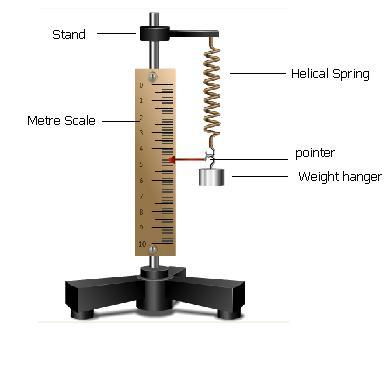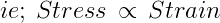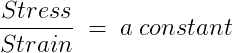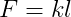Helical Spring

# Objective

To find the force constant of a helical spring by plotting a graph between load and extension.

# Theory

## What is a helical spring?

The helical spring, is the most commonly used mechanical spring in which a wire is wrapped in a coil that resembles a screw thread. It can be designed to carry, pull, or push loads. Twisted helical (torsion) springs are used in engine starters and hinges.

Let’s study how we can use the helical spring to do our experiment.

The helical spring is suspended vertically from a rigid support. The pointer is attached horizontally to the free end ofspring. A metre scale is kept vertically in such a way that the tip of the pointer is over the divisions of the scale; but does not touch the scale.

Helical spring works on the principle of Hooke’s Law. Hooke’s Law states that within the limit of elasticity, stress applied is directly proportional to the strain produced.When a load ‘F’ is attached to the free end of a spring, then the spring elongates through a distance ‘l’ .Here ‘l’ is known as the extension produced. According to Hooke’s Law, extension is directly proportional to the load.

This can be represented as:where ‘k’ is constant of proportionality.  It is called the force constant or the spring constant of the spring.

A graph is drawn with load M in kg wt along X axis and extension, l in metre along the Y axis. The graph is a straight line whose slope will give the value of spring constant, k .

# Learning Outcomes:

•     Students understand the principle of  Hooke’s Law.
•     They learn about the force constant of a spring.
•     Students understand the relationship between force applied and extension produced in a spring.